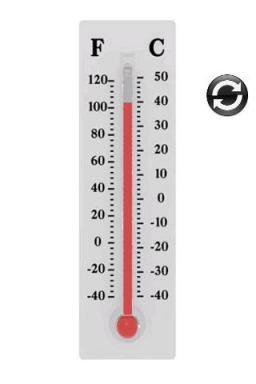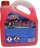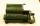# The temperature

The temperature at 1:00 was 10 F. Between 1:00 and 2:00, the temperature dropped 15F. Between 2:00 and 3:00, the temperature rose 3F. What is the temperature at 3:00?

t =  -2 °F

### Step-by-step explanation:

$t=10-15+3=-2\text{°F}$Did you find an error or inaccuracy? Feel free to write us. Thank you!## Related math problems and questions:

• Temperature variationsToday's temperature was 80 degrees, and then the temperature dropped 10 degrees. Then it dropped 15 degrees again, then the next day, the temperature went up 2 degrees. What would the temperature be?In about 12 hours in North Dakota the temperature rose from -33 degrees farenheit to 50 degrees farenheit. By how much did the temperature change?
• The temperature 8The temperature on Monday was –6°C. On Tuesday, the temperature was 3 degrees lower. Write down the temperature on Tuesday.
• LaboratoryMang Elio went to his laboratory at 7:00am And recorded that the temperature was 11.07°C. At lunchtime, the temperature rose by 27.9°C but dropped by 8.05°C at 5:00 PM. What was the temperature at 5:00 PM?
• The temperature 10The temperature in a freezer is -15°C, and it increases by 3°C.
• WeatherWeather. At 5:00 am, the temperature was 28°C, and at noontime, it rose by 9°C. By 4:00 pm, it dropped 3°C, and by nighttime, it was 4°C less. What is the room temperature this time?
• Outside temperatureThe temperature outside was 57 degree Fahrenheit. During the next few hours it decreased by 18 degrees and then increased by 23 degrees. Find new temperature.
• ThermometerThe thermometer showed -12 degrees Celsius in the morning then the temperature rises by 4 degrees and later again increased by 2 degrees at the evening has fallen by 5 degrees and then falls 3 degrees. What end temperature does the thermometer show?
• Evaluate expressionIf x=2, y=-5 and z=3 what is the value of x-2y
• IntegersMay be the sum of two integers less than their difference?
• Expression 8Evaluate this expressions: a) 5[3 + 4(2.8 - 3)] b) 5×(8-4)÷4-2At a weather centre, the temperature at midnight was -2 degree Celsius and by noon it had raised 4 degree Celsius. What is the new temperature?Why does 1 3/4 + 2 9/10 equal 4.65? How do you solve this?The temperature was very cold, then it doubled, then it dropped 10 degrees, and then it increased by 40 degrees. The temperature is now 16 degrees. What was the starting temperature?The temperature at 9 AM is 2 degrees. The temperature rises 3 more degrees by noon. Which expression describes the temperature at noon? Calculate the new temperature value.The temperature at midmorning was 31°C. Early in the evening, the temperature dropped by 5°C. What was the resulting temperature?Evaluate expression: -6-2(4-8)-9